Home Practice
For learners and parents For teachers and schools
Textbooks
Full catalogue
Pricing SupportLog in

We think you are located in United States. Is this correct?

# 11.3 Power and energy

## 11.3 Power and energy (ESBQD)

### Electrical power (ESBQF)

temp text

A source of energy is required to drive current round a complete circuit. This is provided by batteries in the circuits you have been looking at. The batteries convert chemical potential energy into electrical energy. The energy is used to do work on the electrons in the circuit.

Power is a measure of how rapidly work is done. Power is the rate at which the work is done, work done per unit time. Work is measured in joules (J) and time in seconds (s) so power will be $$\frac{\text{J}}{\text{s}}$$ which we call a watt (W).

In electric circuits, power is a function of both voltage and current and we talk about the power dissipated in a circuit element:

Electrical Power

Electrical power is the rate at which electrical energy is converted in an electric circuit. It calculated as:

$P = I·V$

Power (P) is exactly equal to current (I) multiplied by voltage (V), there is no extra constant of proportionality. The unit of measurement for power is the watt (abbreviated W).

It was James Prescott Joule, not Georg Simon Ohm, who first discovered the mathematical relationship between power dissipation and current through a resistance. This discovery, published in 1841, followed the form of the equation: $$P = I^{2}R$$ and is properly known as Joule's Law. However, these power equations are so commonly associated with the Ohm's Law equations relating voltage, current, and resistance that they are frequently credited to Ohm.

#### Equivalent forms

We can use Ohm's Law to show that $$P=VI$$ is equivalent to $$P=I^2R$$ and $$P=\frac{V^2}{R}$$.

Using $$V=I\cdot R$$ allows us to show: \begin{align*} P &= V\cdot I \\ & = (I \cdot R ) \cdot I\ \text{Ohm's Law} \\ & = I^2R \end{align*}

Using $$I=\frac{V}{R}$$ allows us to show: \begin{align*} P &= V\cdot I \\ & = V \cdot \frac{V}{R}\ \text{Ohm's Law} \\ & = \frac{V^2}{R} \end{align*}

## Worked example 8: Electrical power

Given a circuit component that has a voltage of $$\text{5}$$ $$\text{V}$$ and a resistance of $$\text{2}$$ $$\text{Ω}$$ what is the power dissipated?

### Write down what you are given and what you need to find

\begin{align*} V & = \text{5}\text{ V} \\ R & = \text{2}\text{ Ω} \\ P & = ? \end{align*}

### Write down an equation for power

The equation for power is: $P = V^{2}R$

### Solve the problem

\begin{align*} P & = \frac{V^{2}}{R} \\ & = \frac{(\text{5})^{2}}{(\text{2})} \\ & = \text{12,5}\text{ W} \end{align*} The power is $$\text{12,5}$$ $$\text{W}$$.

## Worked example 9: Electrical power

Study the circuit diagram below: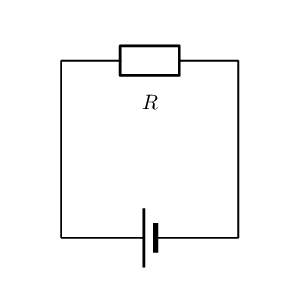The resistance of the resistor is $$\text{15}$$ $$\text{Ω}$$ and the current going through the resistor is $$\text{4}$$ $$\text{A}$$. What is the power for the resistor?

### Determine how to approach the problem

We are given the resistance of the resistor and the current passing through it and are asked to calculate the power. We can have verified that: $P = I^2R$

### Solve the problem

We can simply substitute the known values for $$R$$ and $$I$$ to solve for $$P$$. \begin{align*} P &= I^2R \\ &= (\text{4})^2 \times \text{15} \\ &= \text{240}\text{ W} \end{align*}

The power for the resistor is $$\text{240}$$ $$\text{W}$$.

## Worked example 10: Power in series circuit

Two ohmic resistors ($$R_{1}$$ and $$R_{2}$$) are connected in series with a cell. Find the resistance and power of $$R_{2}$$, given that the current flowing through $$R_{1}$$ and $$R_{2}$$ is $$\text{0,25}$$ $$\text{A}$$ and that the voltage across the cell is $$\text{6}$$ $$\text{V}$$. $$R_{1}$$ = $$\text{1}$$ $$\text{Ω}$$.

### Draw the circuit and fill in all known values.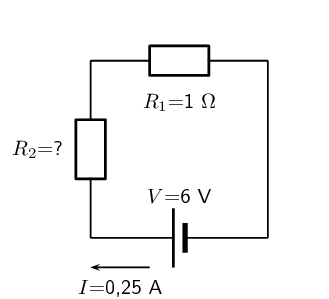### Determine how to approach the problem.

We can use Ohm's Law to find the total resistance R in the circuit, and then calculate the unknown resistance using:

$R = R_{1} + R_{2}$

because it is in a series circuit.

### Find the total resistance

\begin{align*} V &=R\cdot I \\ R &= \frac{V}{I} \\ & =\frac{\text{6}}{\text{0,25}} \\ & = \text{24}\text{ Ω} \end{align*}

### Find the unknown resistance

We know that:

$R = \text{24}\text{ Ω}$

and that

$R_{1} = \text{1}\text{ Ω}$

Since

$R = R_{1} + R_{2}$ $R_{2} = R - R_{1}$

Therefore,

$R = \text{23}\text{ Ω}$

### Solve the problem

Now that the resistance is known and the current, we can determine the power: \begin{align*} P&=I^2R \\ &=(\text{0,25})^2(\text{23}) \\ &=\text{1,44}\text{ W} \end{align*}

The power for the resistor $$R_2$$ is $$\text{1,44}$$ $$\text{W}$$.

temp text

Notice that we use the same circuits in examples as we extend our knowledge of electric circuits. This is to emphasise that you can always combine all of the principles you have learnt when dealing with any circuit.

## Worked example 11: Power in series and parallel networks of resistors

Given the following circuit: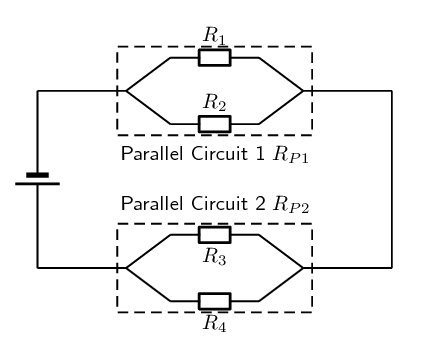The current leaving the battery is $$\text{1,07}$$ $$\text{A}$$, the total power dissipated in the circuit is $$\text{6,42}$$ $$\text{W}$$, the ratio of the total resistances of the two parallel networks $$R_{P1} : R_{P2}$$ is 1:2, the ratio $$R_1 : R_2$$ is 3:5 and $$R_3=\text{7}\text{ Ω}$$.

Determine the:

1. voltage of the battery,
2. the power dissipated in $$R_{P1}$$ and $$R_{P2}$$, and
3. the value of each resistor and the power dissipated in each of them.

### What is required

In this question you are given various pieces of information and asked to determine the power dissipated in each resistor and each combination of resistors. Notice that the information given is mostly for the overall circuit. This is a clue that you should start with the overall circuit and work downwards to more specific circuit elements.

### Calculating the voltage of the battery

Firstly we focus on the battery. We are given the power for the overall circuit as well as the current leaving the battery. We know that the voltage across the terminals of the battery is the voltage across the circuit as a whole.

We can use the relationship $$P=VI$$ for the entire circuit because the voltage is the same as the voltage across the terminals of the battery: \begin{align*} P &=VI \\ V &= \frac{P}{I} \\ &=\frac{\text{6,42}}{\text{1,07}} \\ &= \text{6,00}\text{ V} \end{align*}

The voltage across the battery is $$\text{6,00}$$ $$\text{V}$$.

### Power dissipated in $$R_{P1}$$ and $$R_{P2}$$

Remember that we are working from the overall circuit details down towards those for individual elements, this is opposite to how you treated this circuit earlier.

We can treat the parallel networks like the equivalent resistors so the circuit we are currently dealing with looks like: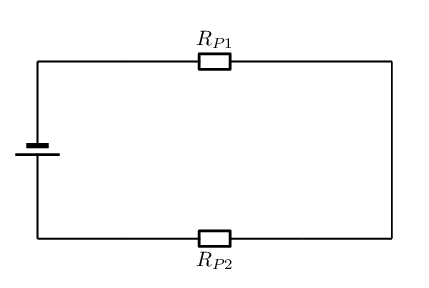We know that the current through the two circuit elements will be the same because it is a series circuit and that the resistance for the total circuit must be: $$R_T=R_{P1}+R_{P2}$$. We can determine the total resistance from Ohm's Law for the circuit as a whole: \begin{align*} V_{battery}&=IR_T \\ R_T &=\frac{V_{battery}}{I} \\ &=\frac{\text{6,00}}{\text{1,07}}\\ &=\text{5,61}\text{ Ω} \end{align*}

We know that the ratio between $$R_{P1} : R_{P2}$$ is 1:2 which means that we know: \begin{align*} R_{P1} &= \frac{1}{2}R_{P2} \ \ \text{and} \\ R_T &= R_{P1} + R_{P2} \\ & = \frac{1}{2}R_{P2} + R_{P2} \\ &=\frac{3}{2}R_{P2} \\ (\text{5,61}) &=\frac{3}{2}R_{P2} \\ R_{P2} &= \frac{2}{3}(\text{5,61}) \\ R_{P2} &= \text{3,74}\text{ Ω} \end{align*} and therefore: \begin{align*} R_{P1} &= \frac{1}{2}R_{P2} \\ &=\frac{1}{2}(\text{3,74}) \\ &= \text{1,87}\text{ Ω} \end{align*}

Now that we know the total resistance of each of the parallel networks we can calculate the power dissipated in each: \begin{align*} P_{P1} &= I^2R_{P1} \\ &= (\text{1,07})^2(\text{1,87}) \\ &= \text{2,14}\text{ W} \end{align*} and \begin{align*} P_{P2} &= I^2R_{P2} \\ &= (\text{1,07})^2(\text{3,74}) \\ &= \text{4,28}\text{ W} \end{align*}

### Parallel network 1 calculations

Now we can begin to do the detailed calculation for the first set of parallel resistors.We know that the ratio between $$R_{1} : R_{2}$$ is 3:5 which means that we know $$R_{1}= \frac{3}{5}R_{2}$$. We also know the total resistance for the two parallel resistors in this network is $$\text{1,87}$$ $$\text{Ω}$$. We can use the relationship between the values of the two resistors as well as the formula for the total resistance ($$\frac{1}{R_PT}=\frac{1}{R_1}+\frac{1}{R_2}$$)to find the resistor values: \begin{align*} \frac{1}{R_{P1}}&=\frac{1}{R_1}+\frac{1}{R_2} \\ \frac{1}{R_{P1}}&=\frac{5}{3R_2}+\frac{1}{R_2} \\ \frac{1}{R_{P1}}&=\frac{1}{R_2}(\frac{5}{3}+1) \\ \frac{1}{R_{P1}}&=\frac{1}{R_2}(\frac{5}{3}+\frac{3}{3}) \\ \frac{1}{R_{P1}}&=\frac{1}{R_2}\frac{8}{3} \\ R_2&=R_{P1}\frac{8}{3} \\ &=(\text{1,87})\frac{8}{3} \\ &=\text{4,99}\text{ Ω} \end{align*} We can also calculate $$R_{1}$$: \begin{align*} R_{1}&= \frac{3}{5}R_{2} \\ &= \frac{3}{5}(\text{4,99}) \\ &= \text{2,99}\text{ Ω} \end{align*}

To determine the power we need the resistance which we have calculated and either the voltage or current. The two resistors are in parallel so the voltage across them is the same as well as the same as the voltage across the parallel network. We can use Ohm's Law to determine the voltage across the network of parallel resistors as we know the total resistance and we know the current: \begin{align*} V &= I R \\ &=(\text{1,07})(\text{1,87}) \\ &=\text{2,00}\text{ V} \end{align*}

We now have the information we need to determine the power through each resistor: \begin{align*} P_1&=\frac{V^2}{R_1} \\ &=\frac{(\text{2,00})^2}{\text{2,99}} \\ &=\text{1,34}\text{ W} \end{align*} \begin{align*} P_2&=\frac{V^2}{R_2} \\ &=\frac{(\text{2,00})^2}{\text{4,99}} \\ &=\text{0,80}\text{ W} \end{align*}

### Parallel network 2 calculations

Now we can begin to do the detailed calculation for the second set of parallel resistors.

We are given $$R_3=\text{7,00}\text{ Ω}$$ and we know $$R_{P2}$$ so we can calculate $$R_4$$ from: \begin{align*} \frac{1}{R_{P2}} &= \frac{1}{R_3}+\frac{1}{R_4} \\ \frac{1}{\text{3,74}} &= \frac{1}{\text{7,00}}+\frac{1}{R_4} \\ R_4&=\text{8,03}\text{ Ω} \end{align*}

We can calculate the voltage across the second parallel network by subtracting the voltage of the first parallel network from the battery voltage, $$V_{P2} = \text{6,00}-\text{2,00}=\text{4,00}\text{ V}$$.

We can now determine the power dissipated in each resistor: \begin{align*} P_3&=\frac{V^2}{R_3} \\ &=\frac{(\text{4,00})^2}{\text{7,00}} \\ &=\text{2,29}\text{ W} \end{align*} \begin{align*} P_4&=\frac{V^2}{R_2} \\ &=\frac{(\text{4,00})^2}{\text{8,03}} \\ &=\text{1,99}\text{ W} \end{align*}

Textbook Exercise 11.6

What is the power of a $$\text{1,00} \times \text{10}^{\text{8}}$$ $$\text{V}$$ lightning bolt having a current of $$\text{2,00} \times \text{10}^{\text{4}}$$ $$\text{A}$$?

\begin{align*} P &=VI \\ &=(\text{1,00} \times \text{10}^{\text{8}})(\text{2,00} \times \text{10}^{\text{4}}) \\ &= \text{2,00} \times \text{10}^{\text{12}}\text{ W} \end{align*}

How many watts does a torch that has $$\text{6,00} \times \text{10}^{\text{2}}$$ $$\text{C}$$ pass through it in $$\text{0,50}$$ $$\text{h}$$ use if its voltage is $$\text{3,00}$$ $$\text{V}$$?

We first need to find the current. Recall from grade 10 that current is charge divided by total time (in seconds):

\begin{align*} I & = \frac{C}{\Delta t} \\ & = \frac{\text{6,00} \times \text{10}^{\text{2}}}{\text{1 800}}\\ & = \text{0,333}\text{ A} \end{align*} \begin{align*} P &=VI \\ &=(\text{3,00})(\text{0,333}) \\ &= \text{0,99}\text{ W} \end{align*}

Find the power dissipated in each of these extension cords:

an extension cord having a $$\text{0,06}$$ $$\text{Ω}$$ resistance and through which $$\text{5,00}$$ $$\text{A}$$ is flowing

\begin{align*} P &=I^{2}R \\ &=(\text{5,00})^{2}(\text{0,06}) \\ &= \text{1,5}\text{ W} \end{align*}

a cheaper cord utilising (using) thinner wire and with a resistance of $$\text{0,30}$$ $$\text{Ω}$$, through which $$\text{5,00}$$ $$\text{A}$$ is flowing

\begin{align*} P &=I^{2}R \\ &=(\text{5,00})^{2}(\text{0,30}) \\ &= \text{7,5}\text{ W} \end{align*}

Determine the power dissipated by each the resistors in the following circuits, if the batteries are $$\text{6}$$ $$\text{V}$$: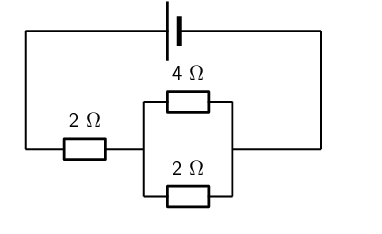We start by determining the equivalent resistance of the parallel combination:

\begin{align*} \frac{1}{R_{p}} & = \frac{1}{R_{1}} + \frac{1}{R_{2}} \\ & = \frac{1}{4} + \frac{1}{2} \\ & = \frac{3}{4}\\ R_{p} & = \text{1,33}\text{ Ω} \end{align*}

Now we have a circuit with two resistors in series so we can calculate the equivalent resistance:

\begin{align*} R_{s} & = R_{3} + R_{p} \\ & = \text{2} + \text{1,33} \\ & = \text{3,33}\text{ Ω} \end{align*}

Now we can calculate the total current:

\begin{align*} I & = \frac{V}{R} \\ & = \frac{6}{\text{3,33}} \\ & = \text{1,8}\text{ A} \end{align*}

This is the current in the $$\text{2}$$ $$\text{Ω}$$ resistor and through the entire parallel connection. Using this we can find the power dissipated in the $$\text{2}$$ $$\text{Ω}$$ resistor:

\begin{align*} P & = I^{2}R \\ & = (\text{1,8})^{2}(\text{2}) \\ & = \text{6,48}\text{ W} \end{align*}

Next we find the voltage across this resistor and use this to find the voltage across the parallel combination:

\begin{align*} V & = IR \\ & = (\text{1,8})(\text{2}) \\ & = \text{3,6}\text{ V} \end{align*} \begin{align*} V_{T} & = V_{1} + V_{2} \\ V_{2} & = V_{T} - V_{1} \\ & = \text{6} - \text{3,6} \\ & = \text{2,4}\text{ V} \end{align*}

This is the voltage across each of the parallel resistors. So we can find the power dissipated by each of these resistors:

\begin{align*} P & = \frac{V^{2}}{R} \\ & = \frac{(\text{2,4})^{2}}{\text{4}} \\ & = \text{1,44}\text{ W} \end{align*} \begin{align*} P & = \frac{V^{2}}{R} \\ & = \frac{(\text{2,4})^{2}}{\text{2}} \\ & = \text{2,88}\text{ W} \end{align*}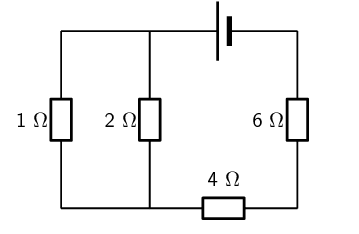We start by determining the equivalent resistance of the parallel combination:

\begin{align*} \frac{1}{R_{p}} & = \frac{1}{R_{1}} + \frac{1}{R_{2}} \\ & = \frac{1}{1} + \frac{1}{2} \\ & = \frac{3}{2}\\ \therefore R_{p} & = \text{0,6666}\ldots\text{ Ω} \end{align*}

Now we have a circuit with three resistors in series so we can calculate the equivalent resistance:

\begin{align*} R_{s} & = R_{3} + R_{4} + R_{p} \\ & = \text{4} + \text{6} + \text{0,6666}\ldots \\ & = \text{10,6666}\ldots\text{ Ω} \end{align*}

Now we can calculate the total current:

\begin{align*} I & = \frac{V}{R} \\ & = \frac{6}{\text{10,6666}\ldots} \\ & = \text{0,5625}\text{ A} \end{align*}

This is the current in the two series resistor and through the entire parallel connection. Using this we can find the power dissipated in the two series resistors:

\begin{align*} P & = I^{2}R \\ & = (\text{0,5625})^{2}(\text{6}) \\ & = \text{1,8984}\ldots\text{ W} \end{align*} \begin{align*} P & = I^{2}R \\ & = (\text{0,5625})^{2}(\text{4}) \\ & = \text{1,2656}\ldots\text{ W} \end{align*}

Next we find the voltage across each of these resistors and use this to find the voltage across the parallel combination:

\begin{align*} V & = IR \\ & = (\text{0,5625})(\text{6}) \\ & = \text{3,375}\text{ V} \end{align*} \begin{align*} V & = IR \\ & = (\text{0,5625})(\text{4}) \\ & = \text{2,25}\text{ V} \end{align*} \begin{align*} V_{T} & = V_{1} + V_{2} + V_{p} \\ V_{p} & = V_{T} - V_{1} - V_{2} \\ & = \text{6} - \text{3,375} - \text{2,25} \\ & = \text{0,375}\text{ V} \end{align*}

This is the voltage across each of the parallel resistors. So we can find the power dissipated by each of these resistors:

\begin{align*} P & = \frac{V^{2}}{R} \\ & = \frac{(\text{0,375})^{2}}{\text{1}} \\ & = \text{0,1406}\ldots\text{ W} \\ &\approx \text{0,14} \text{ W} \end{align*} \begin{align*} P & = \frac{V^{2}}{R} \\ & = \frac{(\text{0,375})^{2}}{\text{2}} \\ & = \text{0,0703}\ldots\text{ W} \\ & \approx \text{0,07} \text{ W} \end{align*}

Also determine the value of the unknown resistor if the total power dissipated is $$\text{9,8}$$ $$\text{W}$$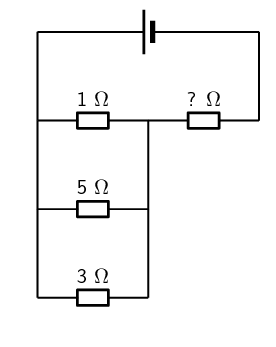We start by calculating the equivalent resistance of the resistors. We know the total power and the total voltage, so we use that to find the total resistance.

\begin{align*} P & = \frac{V^{2}}{R_{T}} \\ R_{T} & = \frac{V^{2}}{P} \\ & = \frac{\text{6}^{2}}{\text{9,8}} \\ & = \text{3,67}\text{ Ω} \end{align*}

We can now find the unknown resistance by first calculating the equivalent parallel resistance:

\begin{align*} \frac{1}{R_{p}} & = \frac{1}{R_{1}} + \frac{1}{R_{2}} + \frac{1}{R_{3}} \\ & = \frac{1}{1} + \frac{1}{5} + \frac{1}{3} \\ & = \frac{23}{15}\\ R_{p} & = \text{0,65}\text{ Ω} \end{align*} \begin{align*} R_{s} & = R_{4} + R_{p} \\ R_{4} & = R_{s} - R_{p} \\ & = \text{3,67} - \text{0,65} \\ & = \text{3,02}\text{ Ω} \end{align*}

Now we can calculate the total current:

\begin{align*} I & = \frac{V}{R} \\ & = \frac{6}{\text{3,67}} \\ & = \text{1,63}\text{ A} \end{align*}

This is the current in the series resistor and through the entire parallel connection. Using this we can find the power dissipated in the series resistor:

\begin{align*} P & = I^{2}R \\ & = (\text{1,63})^{2}(\text{3,02}) \\ & = \text{0,89}\text{ W} \end{align*}

Next we find the voltage across this resistors and use this to find the voltage across the parallel combination:

\begin{align*} V & = IR \\ & = (\text{1,63})(\text{3,02}) \\ & = \text{4,92}\text{ V} \end{align*} \begin{align*} V_{T} & = V_{1} + V_{p} \\ V_{p} & = V_{T} - V_{1} \\ & = \text{6} - \text{4,92} \\ & = \text{1,08}\text{ V} \end{align*}

This is the voltage across each of the parallel resistors. So we can find the power dissipated by each of these resistors:

\begin{align*} P & = \frac{V^{2}}{R} \\ & = \frac{(\text{1,08})^{2}}{\text{1}} \\ & = \text{1,17}\text{ W} \end{align*} \begin{align*} P & = \frac{V^{2}}{R} \\ & = \frac{(\text{1,08})^{2}}{\text{5}} \\ & = \text{5,83}\text{ W} \end{align*} \begin{align*} P & = \frac{V^{2}}{R} \\ & = \frac{(\text{1,08})^{2}}{\text{3}} \\ & = \text{3,5}\text{ W} \end{align*}

Examine the circuit below: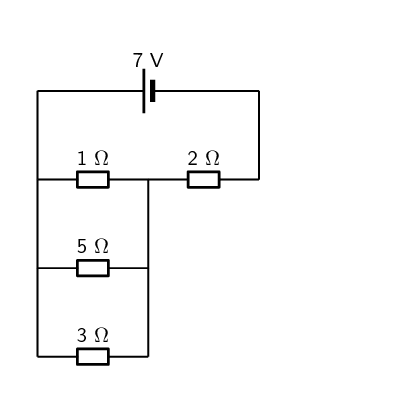If the potential difference across the cell is $$\text{7}$$ $$\text{V}$$, calculate:

the current $$I$$ through the cell.

We can find the equivalent parallel resistance:

\begin{align*} \frac{1}{R_{p}} & = \frac{1}{R_{1}} + \frac{1}{R_{2}} + \frac{1}{R_{3}} \\ & = \frac{1}{1} + \frac{1}{5} + \frac{1}{3} \\ & = \frac{23}{15}\\ R_{p} & = \text{0,65217}\ldots\text{ Ω} \end{align*}

And now we can find the equivalent resistance:

\begin{align*} R_{s} & = R_{4} + R_{p} \\ & = \text{2} + \text{0,65217}\ldots \\ & = \text{2,65217}\ldots\text{ Ω} \end{align*}

Now we can calculate the total current:

\begin{align*} I & = \frac{V}{R} \\ & = \frac{7}{\text{2,65217}\ldots} \\ & = \text{2,63934}\ldots\text{ A} \\ & \approx \text{2,64 A} \end{align*}

the current through the $$\text{5}$$ $$\Omega$$ resistor

For a series circuit the total current is equal to the current in each of the resistors. Since we can consider the total parallel combination as one series connection the total current through the connection is $$\text{2,64}$$ $$\text{A}$$.

Using this and the total parallel resistance we can calculate the voltage across each resistor:

\begin{align*} V & = IR \\ & = (\text{2,63934}\ldots)(\text{0,65217}\ldots) \\ & = \text{1,72131}\ldots\text{ V} \end{align*}

Now we can calculate the current through the $$\text{5}$$ $$\Omega$$ resistor:

\begin{align*} I & = \frac{V}{R} \\ & = \frac{\text{1,72131}\ldots}{\text{5}} \\ & = \text{0,34426}\ldots\text{ A} \\ & \approx \text{0,34 A} \end{align*}

the power dissipated in the $$\text{5}$$ $$\Omega$$ resistor

We know the resistance, the voltage and the current for this resistor so we can use any two of these to find the power. We will use the resistance and the current:

\begin{align*} P & = I^{2}R \\ & = (\text{0,34426}\ldots)^{2}(\text{5}) \\ & = \text{0,59258}\ldots\text{ W} \\ & \approx \text{0,59 W} \end{align*}

If current flowing through the cell is $$\text{2}$$ $$\text{A}$$, and all the resistors are ohmic, calculate the power dissipated in each of the resistors, $$R_1$$, $$R_2$$, and $$R_3$$ respectively.We start by determining the equivalent resistance of the parallel combination:

\begin{align*} \frac{1}{R_{p}} & = \frac{1}{R_{2}} + \frac{1}{R_{3}} \\ & = \frac{1}{4} + \frac{1}{2} \\ & = \frac{3}{4}\\ R_{p} & = \text{1,33}\text{ Ω} \end{align*}

Now we have a circuit with two resistors in series so we can calculate the equivalent resistance:

\begin{align*} R_{s} & = R_{1} + R_{p} \\ & = \text{4,66} + \text{1,33} \\ & = \text{5,99}\text{ Ω} \end{align*}

We are given the total current and this is the current in the series resistor and through the entire parallel connection. Using this we can find the power dissipated in the series resistor:

\begin{align*} P & = I^{2}R \\ & = (\text{2})^{2}(\text{4,66}) \\ & = \text{18,64}\text{ W} \end{align*}

Next we find the voltage across this resistor:

\begin{align*} V & = IR \\ & = (\text{2})(\text{4,66}) \\ & = \text{9,32}\text{ V} \end{align*}

And we use the total resistance and the total current to find the total voltage:

\begin{align*} V & = IR \\ & = (\text{2})(\text{5,99}) \\ & = \text{12}\text{ V} \end{align*}

Now we can find the voltage across the parallel combination of resistors:

\begin{align*} V_{T} & = V_{1} + V_{p} \\ V_{p} & = V_{T} - V_{1} \\ & = \text{12} - \text{9,32} \\ & = \text{2,68}\text{ V} \end{align*}

This is the voltage across each of the parallel resistors. So we can find the power dissipated by each of these resistors:

\begin{align*} P & = \frac{V^{2}}{R} \\ & = \frac{(\text{2,68})^{2}}{\text{4}} \\ & = \text{10,72}\text{ W} \end{align*} \begin{align*} P & = \frac{V^{2}}{R} \\ & = \frac{(\text{2,68})^{2}}{\text{2}} \\ & = \text{5,36}\text{ W} \end{align*}

### Electrical energy (ESBQG)

temp text

When power is dissipated in a device there is a transfer of energy from one kind to another. For example, a resistor may get very hot which indicates that the energy is being dissipated as heat. Power was the rate at which work was done, the rate at which energy is transferred. If we want to calculate the total amount of energy we need to multiply the rate of energy transfer by the time over which that energy transfer took place.

Electrical energy is simply power times time. Mathematically we write: $E = P \times t$ Energy is measured in joules (J) and time in seconds (s).

## Worked example 12: Electrical energy

A $$\text{30}$$ $$\text{W}$$ light bulb is left on for $$\text{8}$$ hours overnight, how much energy was wasted?

### What is required

We need to determine the total amount of electrical energy dissipated by the light bulb. We know the relationship between the power and energy and we are given the time. Time is not given in the correct units so we first need to convert to S.I. units: \begin{align*} \text{8}\text{ hr}&= 8 \times \text{3 600}\text{ s} \\ &=\text{28 800}\text{ s} \end{align*}

### Calculate the energy

We know that: \begin{align*} E&=Pt \\ &=(\text{30})(\text{28 800})\\ &=\text{864 000}\text{ J} \end{align*}

## Worked example 13: Electrical energy

Study the circuit diagram below:The resistance of the resistor is $$\text{27}$$ $$\text{Ω}$$ and the current going through the resistor is $$\text{3,3}$$ $$\text{A}$$. What is the power for the resistor and how much energy is dissipated in $$\text{35}$$ $$\text{s}$$?

### Determine how to approach the problem

We are given the resistance of the resistor and the current passing through it and are asked to calculate the power. We have verified that: $P = I^2R$ and we know that $E = Pt$

### Solve the problem

We can simply substitute the known values for $$R$$ and $$I$$ to solve for $$P$$. \begin{align*} P &= I^2R \\ &= (\text{3,3})^2 \times \text{27} \\ &= \text{294,03}\text{ W} \end{align*}

Now that we have determined the power we can calculate the energy:

\begin{align*} E &= Pt \\ &= (\text{294,03})(\text{35}) \\ &= \text{10 291,05 J} \end{align*}

The power for the resistor is $$\text{294,03}$$ $$\text{W}$$ and $$\text{10 291,05}$$ $$\text{J}$$ are dissipated.

Electricity is sold in units which are one kilowatt hour ($$\text{kWh}$$). A kilowatt hour is simply the use of $$\text{1}$$ $$\text{kW}$$ for $$\text{1}$$ $$\text{hr}$$. Using this you can work out exactly how much electricity different appliances will use and how much this will cost you.

## Worked example 14: Cost of electricity

How much does it cost to run a $$\text{900}$$ $$\text{W}$$ microwave oven for $$\text{2,5}$$ $$\text{minutes}$$ if the cost of electricity is $$\text{61,6}$$ $$\text{c}$$ per $$\text{kWh}$$?

### What is required

We are given the details for a device that uses electrical energy and the price of electricity. Given a certain amount of time for use we need to determine how much energy was used and what the cost of that would be.

The various quantities provided are in different units. We need to use consistent units to get an answer that makes sense.

The microwave is given in $$\text{W}$$ but we can convert to $$\text{kW}$$:$$\text{900}\text{ W}=\text{0,9}\text{ kW}$$.

Time is given in minutes but when working with household electricity it is normal to work in hours. $$\text{2,5}\text{ minutes} = \frac{\text{2,5}}{\text{60}} = \text{4,17} \times \text{10}^{-\text{2}}\text{ h}$$.

### Calculate usage

The electrical power is: \begin{align*} E&=Pt \\ &=(\text{0,9} ) (\text{4,17} \times \text{10}^{-\text{2}}) \\ &=\text{3,75} \times \text{10}^{-\text{2}}\text{ kWh} \end{align*}

### Calculate cost (C) of electricity

The cost for the electrical power is: \begin{align*} C&=E \times \text{price} \\ &=(\text{3,75} \times \text{10}^{-\text{2}} ) (\text{61,6}) \\ &=\text{3,75} \times \text{10}^{-\text{2}}\text{ kWh} \\ &=\text{2,31}\text{ c} \end{align*}

temp text

## Using electricity

The following table gives the cost of electricity for users who consume less than $$\text{450}$$ $$\text{kWh}$$ on average per month.

 Units ($$\text{kWh}$$) Cost per unit ($$\text{c}$$) $$\text{0}$$–$$\text{150}$$ $$\text{61,60}$$ $$\text{150}$$–$$\text{350}$$ $$\text{81,04}$$ $$\text{350}$$–$$\text{600}$$ $$\text{107,43}$$ $$>$$ $$\text{600}$$ $$\text{118,06}$$

You are given the following appliances with their power ratings.

 Appliance Power rating Stove $$\text{3 600}$$ $$\text{W}$$ Microwave $$\text{1 200}$$ $$\text{W}$$ Washing machine $$\text{2 200}$$ $$\text{W}$$ Kettle $$\text{2 200}$$ $$\text{W}$$ Fridge $$\text{230}$$ $$\text{W}$$ Toaster $$\text{750}$$ $$\text{W}$$ Energy saver globe $$\text{40}$$ $$\text{W}$$ Light bulb $$\text{120}$$ $$\text{W}$$ Vacuum cleaner $$\text{1 600}$$ $$\text{W}$$

You have $$\text{R}\,\text{150,00}$$ to spend on electricity each month.

1. Which usage class do you fall into?

2. Complete the following table.

 Appliance Cost to run for 1 hour Stove Microwave Washing machine Kettle Fridge Toaster Energy saver globe Vacuum cleaner
3. For how long can you use each appliance to ensure that you spend less than $$\text{R}\,\text{150,00}$$ per month? Assume you are using a maximum of 20 energy saver globes around your home.